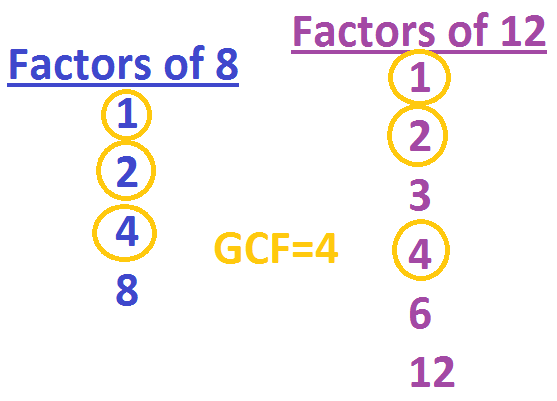# Reducing Fractions: Rules & Practice

Instructor: Allison Petrovic

Allison has experience teaching high school and college mathematics and has a master's degree in mathematics education.

In this lesson, we will learn how to reduce fractions by using an example with M&M's! We will see how important the greatest common factor is when reducing fractions.

## Delicious M&M's!

M&M's are one of my favorite candies. As I open my bag of M&M's, I count 25 pieces of candy. Counting by color, I have 10 blue M&M's. I want to create a fraction to show how many blue M&M's that I have. I remember that a fraction needs a numerator and a denominator. The numerator, number on top, tells us how many parts out of the whole that we have. The denominator, number on the bottom, tells us how many we have as a whole.

When setting up a fraction to show how many blue M&M's I have, the number of blue M&M's is the numerator. So the numerator would be 10. The total number of M&M's is the denominator. The denominator would be 25. My fraction of blue M&M's would be 10/25. The fraction 10/25 can be reduced! We need to go over one definition before we learn the steps to reducing fractions.

## Greatest Common Factor

Remember that a factor is a number that goes into another number evenly. Look at the number 8. We know that 2x4=8. We can say that the numbers 2 and 4 are both factors of 8.

The greatest common factor is the largest number that goes into two numbers. Look at the numbers 8 and 12. We first need to look at all of the factors of both numbers. Then we can find the largest factor that they have in common!

Look at 8 first. We know that 1 x 8 = 8 and 2 x 4 = 8. So the factors of 8 are 1, 2, 4, and 8. Now let's look at 12. We know that 1 x 12 = 12, 2 x 6 = 12, and 3 x 4 = 12. So the factors of 12 are 1, 2, 3, 4, 6, and 12.

Now we have to see what factors they have in common. Looking at our two lists of factors, both numbers have factors of 1, 2, and 4. Now we just find the greatest of the common factors, and we have the greatest common factor! So we can say that 4 is the great common factor of 8 and 12!## How to Reduce Fractions

Now that you can find the greatest common factor, you can use just two easy steps to reduce a fraction.

To unlock this lesson you must be a Study.com Member.

### Register to view this lesson

Are you a student or a teacher?

#### See for yourself why 30 million people use Study.com

##### Become a Study.com member and start learning now.
Back
What teachers are saying about Study.com

### Earning College Credit

Did you know… We have over 200 college courses that prepare you to earn credit by exam that is accepted by over 1,500 colleges and universities. You can test out of the first two years of college and save thousands off your degree. Anyone can earn credit-by-exam regardless of age or education level.